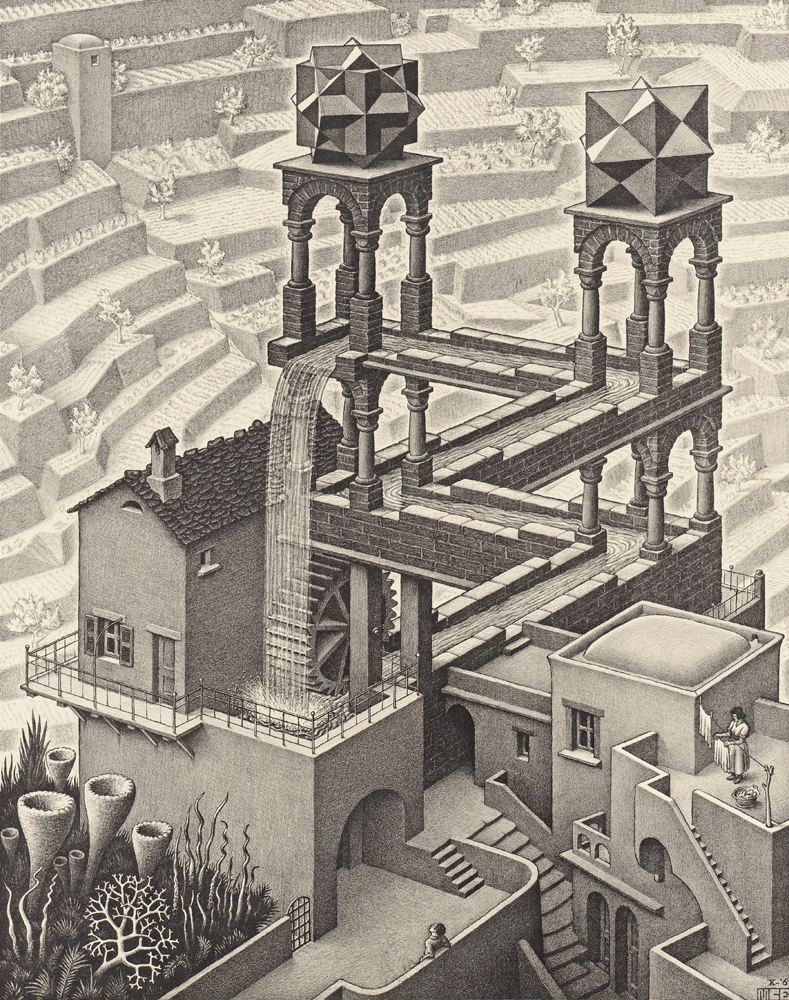# The Well-known MU Puzzle

Logic Level 3In Douglas Hofstadter's book, Godel, Escher, Bach, he proposes the following puzzle about formal systems.

Axiom: MI is an axiom.

Theorem: The axiom is a theorem by default. All the (and only all the) strings that can be derived using the following transformations on a theorem is also a theorem.

 Formal Rule Informal Description Example xI → xIU Changing any terminal I to IU MII → MIIU Mx → Mxx Double the string after M MIU → MIUIU xIIIy → xUy Replace three Is with an U MIIIU → MUU xUUy → xy Remove an occurence of double Us MUUI → MI

Problem: Is MU a theorem?

Guessing the answer to this problem is not very difficult. However, an interested problem solver could explore this questions:

• If your answer was yes, what is the proof of the MU theorem, i.e, what is the sequence of transformation rules that will take you from MI to MU?
• Is the MIU system decidable, i.e, is there a computer program to decide if a given string is a theorem?
• If not, is the system semidecidable, i.e, is there a computer program that will eventually tell if a string is a theorem, but never tell if it isn't?
×